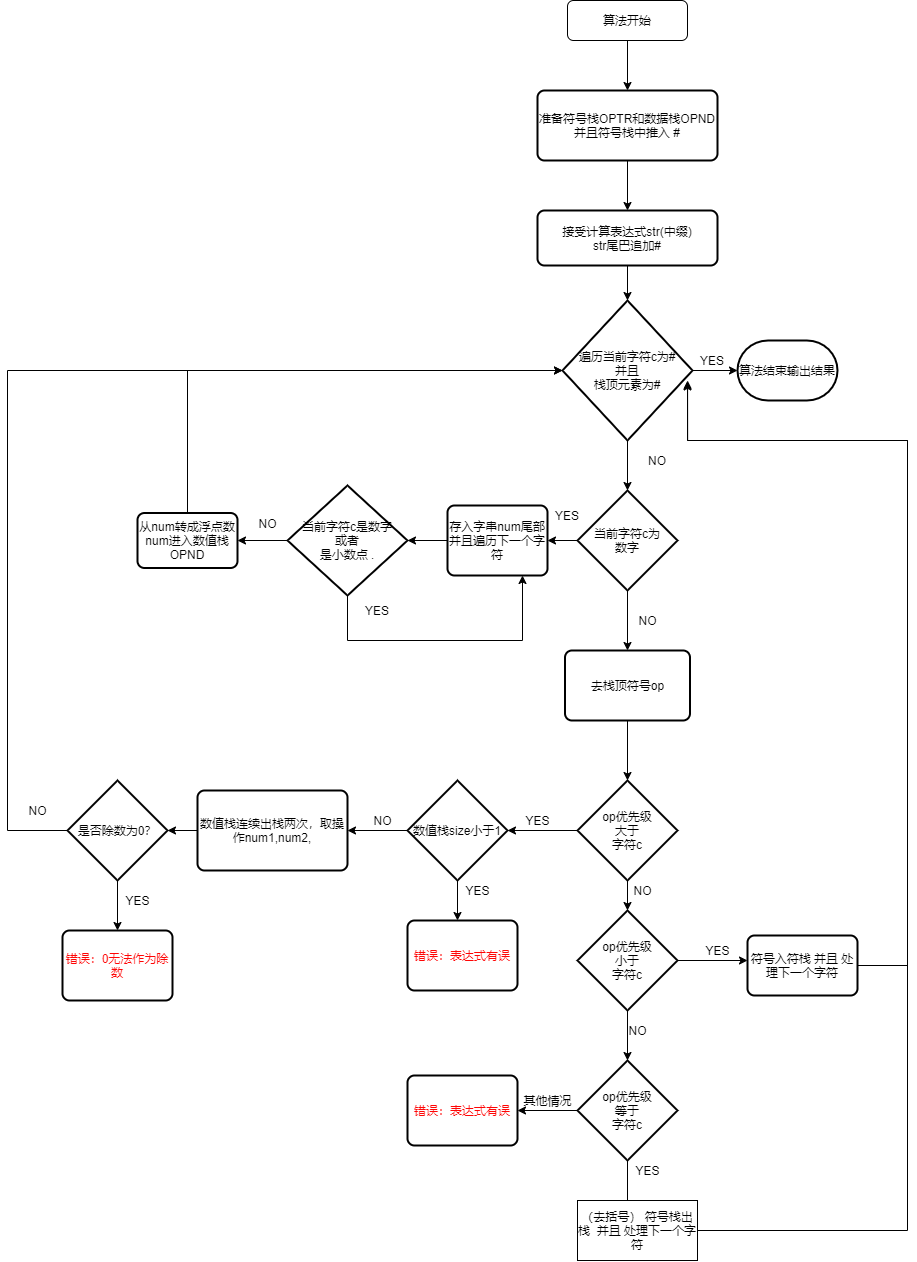## 使用栈进行表达式计算

### 中缀表达式和后缀表达式的转换

1. 逐个按中缀表达式的顺序处理每一个元素（符号和数）

2. 遇到数值直接入追加到后缀表达式的尾部

3. 遇到符号跟当前栈顶元素（当栈为空时，那么当前符号优先级大），如果栈顶符号优先级大，符号出栈追加到后缀表达式的尾，反之追加到后缀表达式的尾

4. 重复123的过程直到中缀表达式字串处理完毕

### 优先级的表达

****计算先从左计算到右****：这句话可以描述成，****同一个运算符优先级也分左右，右运算符大于左运算符（相对操作数左右而言）****，比如1+2+3 ，我们是先计算1+1呢还是先计算2+3？答案是先计算1+2，为啥？其实这里旧隐藏了一个问题，同一个运算符也分左右，1+2中的+优先级比2+3中的高。(注：这里严格按照从左到右计算，无视交换律和结合律)

****去括号，先处理括号内部的运算****：其实这里也好理解，****让左括号的优先级比任何符号的优先级别高，同时让左括号的优先级小于右括号****，这样就可以优先计算括号内部的了。但是这里仅仅做到了优先计算括号内的，但是括号有没有对应的计算，我们需要将它去除。****其实将括号作为一个特例，左括号和右括号优先级相等，并且左括号不进入符号栈****，这样如果我们栈顶元素是右括号的时候，表明括号内部的已经优先处理，所以’(’出栈，不追加到后缀表达式尾。

const char Prior = {
//   +    -   *    /  (   )   #
{'>','>','<','<','<','>','>'},//+
{'>','>','<','<','<','>','>'},//-
{'>','>','>','>','<','>','>'},//*
{'>','>','>','>','<','>','>'},// /
{'<','<','<','<','<','=','$'},//( {'>','>','>','>','$','>','>'},//)
{'<','<','<','<','<','\$','='}// #
};


### 实际流程

- ****栈顶符号优先级大****： 新的操作符 没有旧的运算符高的时候 ，数据栈出栈提取两个操作数 进行旧的操作符运算 并且结果入数据栈 新符号入栈

- *栈顶符号优先级小* 符号入符栈

- *栈顶符号优先级等* 两个括号相遇 去括号 或者 表达运算结束

- 当栈顶和遍历符号都为# 表达计算完成

- 错误处理### 具体代码

double expreetor(std::string str)throw (Exception)
{
stack<int> OPTR; //符号栈
stack<double> OPND;// 数值栈
OPTR.push(isOperator('#'));//符号栈存入起始符
str.push_back('#');//插入截至符号
double result = 0; //结果
int i = 0, op1 = -1, op2 = -1;//遍历字串 和 新操作符 旧操作符
char c = str;
double num1 = 0, num2 = 0;//操作数
std::string num;
while (c != '#'||OPTR.top()!=6)//表达没有结束
{
if (c >= '0' && c <= '9')//得到操作数
{
while ((c >= '0' && c <= '9') || c == '.')
{
num.push_back(c);
c = str[++i];
}
OPND.push(atof(num.c_str()));
num.clear();
}
else//对符号进行处理
{
//取出操作符
op1 = isOperator(c);
op2 = OPTR.top();
switch (pritor(op2, op1))
{
case '<'://符号入符栈 并且 处理下一个字符
OPTR.push(op1);
c = str[++i];
break;
case '='://取括号 符号栈出栈  并且 处理下一个字符
OPTR.pop();
c = str[++i];
break;
case '>'://数据栈出栈提取两个操作数  进行旧的操作符运算  并且结果入数据栈 新符号入栈
if (OPND.size() < 2 || OPTR.size() < 1)
{
Exception e("表示式有误\n");
throw e;
}
num1 = OPND.top();
OPND.pop();
num2 = OPND.top();
OPND.pop();
op2 = OPTR.top();
OPTR.pop();
result = cctor(op2, num2, num1);//注意！！num2 / - num1 取出的顺序式反的
OPND.push(result);
break;
default:
Exception e("表示式有误\n");
throw e;
break;
}//switch
}//else
}
return OPND.top();
}


Q.E.D.